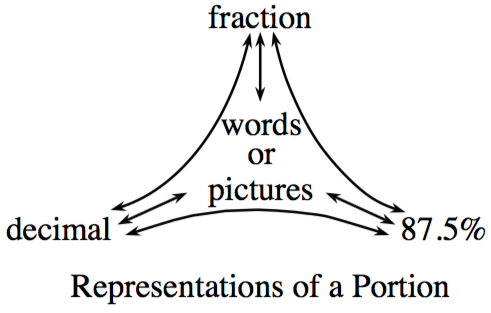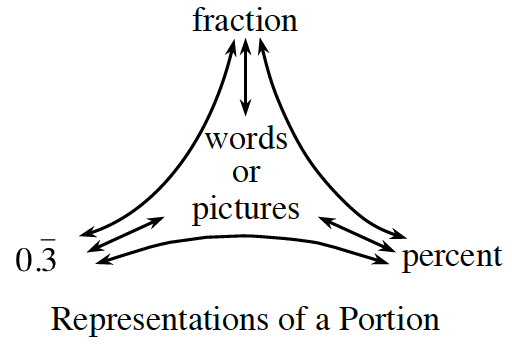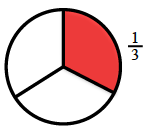### Home > CC1 > Chapter 9 > Lesson 9.2.2 > Problem9-48

9-48.

Complete each of the webs below.

1.Refer to the Math Notes box below if you need help remembering how to do this.

$0.875$

 Decimal to percent:Multiply the decimal by $100$.$\left(0.34\right)\left(100\right)=34\%$Fraction to percent:Set up an equivalent fraction using $100$ as the denominator. The numerator is the percent. $\frac{4}{5}=\frac{80}{100}=80\%$Decimal to fraction: Use the digits as the numerator. Use the decimal place value as the denominator. Simplify as needed. $0.48=\frac{48}{100}=\frac{12}{25}$ Percent to decimal: Divide the percent by $100$. $78.6\%=78.6\div100=0.786$Percent to Fraction: Use $100$ as the denominator. Use the digits in the percent as the numerator. Simplify as needed. $22\%=\frac{22}{100}=\frac{11}{50}$Fraction to decimal: Divide the numerator by the denominator. $\frac{3}{8}=3\div8=0.375$

b.